# Example Models and Libraries

(diff) ← Older revision | Latest revision (diff) | Newer revision → (diff)

If you have installed Analytica, you will find these example models and libraries of useful functions in folders already on your computer. These example models cover a wide variety of application domains, from finance, engineering, economics, to ecology -- and a wide variety of modeling techniques, from Monte Carlo simulation, systems dynamics, risk analysis, statistics, and optimization. They include the models used as examples in this Tutorial and the User Guide . You may find it useful to look through them to find models that show you ways to address your own challenges. They may provide a useful starting point or inspire your own approach.

You can find more example models, not installed with Analytica at Example Models. You can download and fire up these models in Analytica just by clicking on the Download links. These example models were created by a wide variety of Analytica users. If you have models you would like to share with the Analytica community, we invite you to upload them into this wiki yourself. See how at Uploading Example Models

To access the Example Models folder from inside Analytica,

1. Select FileOpen,
2. Press the Example Models button at the top left: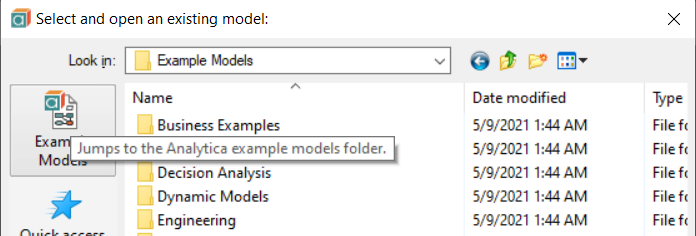The Example Models folder is organized into subfolders, which are the sections in this page:

Bond Model: This model takes typical bond purchase inputs (purchase price, par value, interest rate, and life to maturity) and calculates bond cash flows, current yield, and yield to maturity.

Breakeven Analysis: This model is an example of a breakeven analysis of a set of revenue levels, when the fixed expenses are set at one amount and the variable expenses are a constant fraction of revenue.

Expected R&D Project Value: This model evaluates and compares the expected commercialization value of multiple proposed R&D projects.

Financial Statement Templates: This model contains a complete set of standard financial statements: a profit and loss statement, balance sheet, and cash flow statement. It provides a step-by-step guide to using these templates to generate your own financial statements. You can enter values into the existing template and modify the variable definitions to reflect your own accounting standards.

Market Model: This model explores a market for a new product, and the pricing and advertising budget decisions involved. This example also shows the use of “forms” for receiving input and presenting output for users of the model.Plan_Schedule_ Control: This model takes input data for activity paths required to complete a project, and calculates various outputs describing the critical path, timing, and costs for project completion.

Project Portfolio Planner: This model evaluates and prioritizes a portfolio of projects based on either the estimated net present value or a multi-attribute score, based on strategy fit, staff development, the generation of public goodwill, and estimated net revenue.

Sales Effectiveness: This model evaluates the effects of unit price on salesmen head count and production capacity. The model contains an example of taking user estimates of uncertainty in a standard high- medium-low form, and transforming those inputs into a continuous distribution for propagation through the model. Derived from Principles of Systems by Jay W. Forrester, 1968, ISBN 0-915299-87-9.

Subscriber Pricing: This model determines the amount of revenue needed on a monthly basis from each subscriber of a service to just meet the weighted average cost of capital of the firm from the service release date to the end of the study horizon. In other words, it calculates the monthly unit revenue rate required from each subscriber of a service to give a return on investment at the end of the study horizon that is equal to the weighted average cost of capital of the firm.

Waterfall chart: Demonstrates the use of a waterfall chart to visualize the components of an earnings stream from an asset.

## Data Analysis

Bootstrapping: Bootstrapping is a technique for estimating the confidence in a statistical estimator. This model demonstrates how a distribution for an estimated statistic can be obtained by resampling repeatedly.

Kmeans Clustering: This model shows an example of scatter plots in Analytica. A K-means clustering algorithm (where K is the number of clusters) is applied to some random data to partition points into groups (clusters) of similar points. This model also demonstrates the Iterate function.

Logistic regression prior selection: This model demonstrates the use of Logistic and Probit regression to fit a generalized linear model to breast cancer data. The model demonstrates the impact of the Bayesian prior on generalization performance of Logistic and ProbIt regression.

Moving Average Example: This is a simple model that shows how to compute the moving average for a data stream. It defines a Moving Average function you can use.

Multidimensional Scaling: This model performs multidimensional scaling. It takes as input N, which is the dimensionality of the problem, and Distances, which is an NxN symmetric matrix of distances (or dissimilarities). It calculates and outputs a two-dimensional set of N points XY (or separately as Xcoord and Ycoord) that best approximates the spatial layout of points that could generate the input distances. Reference: Multivariate Analysis by K.V. Mardia, J.T. Kent, and J.M. Bibby, Academic Press, London, 1979, Section 14.2.2, page 400. Model supplied by Michael L. Thompson.

Principle Components : Principal components analysis (PCA) is a technique used to reduce multidimensional data sets to lower dimensions for analysis. PCA involves computing the eigenvalue decomposition or singular value decomposition of a data set. This model shows how to find the principle components using an eigenvalue decomposition to compute the principle components of the covariance matrix of historical stock prices.

Regression Examples: This model demonstrates the use of generalized linear regression by best fit curves of various function forms to a set of (x, y) points. It includes:

• Linear regression
• Polynomial regression
• Discrete Fourier series
• Regression with redundant basis
• Regression using a large arbitrary collection of terms (useful in the situation where you do not have any reason to prefer one functional form over another)
• An auto-regressive series

## Decision Analysis

This folder includes models that illustrate the discipline of decision analysis.

Two Branch Party Tree: The author of this model wants to throw a party, and can’t decide where to throw it. This model shows how to model a two-branch decision tree in Analytica.Beta Updating: This model uses the beta distribution for the Bayesian update of beliefs about the probability that a coin will come up heads.

Biotech R&D Portfolio: This multi-project R&D evaluation models a typical R&D decision problem that might be faced by a biogenetic company.

Diversification Illustration: This model is an example of a Blitzogram™, which is one way to display the effect of diversifying over a growing set of investments. The example from the model is taken from “Blizograms - Interactive Histograms” in Informs Transactions on Education, Vol. 1., No. 2 (Jan. 2001) by Sam Savage. For further information, consult:

Expected Value of Sampling Information (EVSI): The EVSI for further treatment trials model computes the EVSI, a measure of how much value would be obtained from a sample of observations prior to making a decision. In this model, a drug approval agency must decide whether to approve a certain new treatment. They have the option of requesting additional clinical trials before making the decision. The EVSI captures the expected value of the information that would be obtained from the additional trials.

Gibbs Sampling in Bayesian Network: This model solves a Bayesian network using the Gibbs sampling method, also referred to as Stochastic Simulation. It is an instance of Markov Chain Monte Carlo simulation. This implementation runs multiple simulations simultaneously. You can specify observations for any subset of variables in the model (using the pull-down menus), and compute the posterior probabilities for any of the other variables. For more on this technique, see S. L. Lauritzen and D. J. Spiegelhalter, “Local Computations with Probabilities on Graphical Structures and Their Application to Expert Systems,” Journal Royal Statistical Society Series B50:2, 1984, p. 157-224.

LEV R&D Strategy: This example models R&D decision analysis for investment strategy among several choices of powerplants for a low emissions vehicle (LEV).

Marginal Analysis for Control of SO2 Emissions: This model demonstrates a marginal benefit/cost analysis to determine the policy alternative that leads to the most economically efficient level of cleanup.

Multi-attribute Utility Analysis: This model is an example of a multi-attribute utility analysis for cars, showing how to analyze an array of cars across an array of attributes, where different drivers assign differing weights to the importance of each attribute.

Newton-Raphson Method: This model implements the Newton-Raphson (or simply Newton’s) method, one of the most powerful and well-known numerical methods for finding the root of f(x) = 0.

Nonsymmetric Tree: This model uses decision tree terminology to provide an example asymmetric decision tree in Analytica.

Party With Forecast: This model presents a problem facing a party host. In the face of uncertain weather, what is the best location to hold a party? The value the host assigns to the party is a function of both the location chosen and the weather outcome. This model augments the basic party model in order to show the value of imperfect information — in this case, a weather forecast — using Bayesian updating.

Plane catching decision with EVIU: The Expected Value of Including Uncertainty (EVIU) expresses the improvement in decision quality obtained when we include uncertainty as probability distributions in our model, compared to using only deterministic estimates for parameters. This model illustrates how EVIU is computed using a model to assess what time I should leave my home to catch a flight at the airport.

Probability of Gaussian Region (Importance Sampling): This model demonstrates the technique of importance sampling. Importance sampling is a variant of Monte Carlo sampling that can be used for extremely rare event sampling. Straight Monte Carlo with finite sample sizes will provide very low coverage of regions with very low probability. This example demonstrates by estimating the probability of a very small region within a Gaussian distribution.

Supply and Demand: This model calculates the required supply level to maximize profit when the profit function is asymmetric around the average demand value.

Tornado Diagrams: A tornado diagram is a common tool used to depict the sensitivity of a result to changes in selected variables. The fundamental analysis behind a tornado diagram consists of varying only one input variable at a time, keeping all other variables at their nominal values. Typically, a low and a high value are selected for each input, and the output variable is computed while only one variable varies at a time. This example model shows two methods for selecting high and low values: 1. By varying all inputs by the same relative amount, e.g., low=90% of nominal, high=110% of nominal, or 2. By varying all inputs between two given fractiles. This only makes sense if your inputs are uncertain variables. Example: low=10% fractile, high=90% fractile, nominal=50% fractile.Upgrade Decision: This model represents a decision often faced in today’s world: which technology to purchase now, in the face of uncertain future products and prices.## Dynamic Models

This folder includes models that use Analytica’s Dynamic function for performing dynamic simulation, also known as Systems Dynamics -- that is, models with cyclic dependencies or feedback loops.

Disease establishment: This model forecasts the population of fish and the establishment of a contagious viral disease within the population over time.

Leveling: This example levels staff efforts over time according to staff available, computing both the work done over time and idle time.

Markov Chain: This model demonstrates how to simulate a Markov process using dynamic time. The example estimates the number of hospital patients over time, modeled as a Markov process.

Mass-Spring-Damper: This model simulates a typical free mass-spring-damper system. The term “free system” means that there is no time-dependent driving force or displacement acting on the mass. Ordinarily solutions to such a system are determined from a set of homogeneous second-order differential equations accompanied by the appropriate initial conditions. In this model, the kinematic variables (displacement, velocity, and acceleration) are related to the typical kinematic equations, and the dynamic variables (spring force and damper force) are related to the acceleration and the system mass by Newton’s second law. You input the various initial state conditions (spring constant, damper constant, mass, initial displacement, and initial velocity) and the run time of the model. The graphical solutions generated by this dynamic model are comparable to the solutions determined by the corresponding differential equations.

Mean-reversion trading.ana: Models a mean-reverting price process, along with a trading strategy to "beat the market". It demonstrates the encoding of a Markov Decision Process.

Minimal edit distance, a 2-D dynamic: An implementation of the Wagner-Fisher algorithm for computing the Levenshtein distance, which is the minimum number of character edits required to convent one text string into another. The model demonstrates a 2-D dynamic algorithm, where each cell depends on previous cells in two dimensions (i.e., each cell depends on the cell to the left, the cell above, and the cell to the upper-left).

Optimal Path Dynamic Programming: This model demonstrates a simple dynamic programming solution to a sequential decision problem. It computes the optimal finite-time step path to take in a deterministic environment where a known reward payout is given in the final time step and each transition has a known cost.

Parking Space Selection: A simple dynamic programming example, illustrating the solution to a sequential decision problem with uncertainty. You are to find the best parking space to minimize the time needed to get to your restaurant. The example demonstrates the use of the Dynamic() function over an index other than Time and the use of a reverse-recurrence in which the dynamic recurrence is based on future (rather than past) values.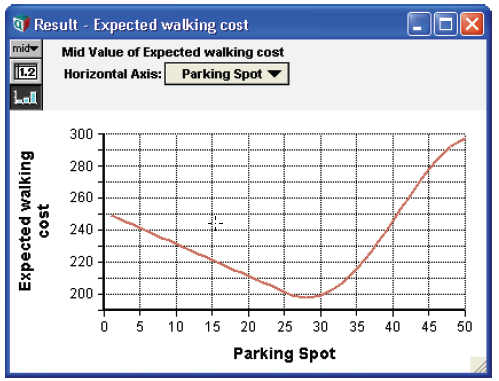Projectile Motion: An example demonstrating how to use the system variable Time and the Dynamic() function to model time-variant behavior — in this case, the motion of a projectile.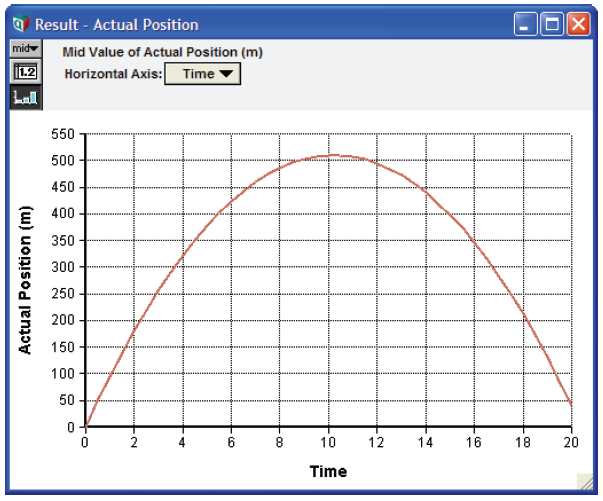Tunnel through earth: Imagine a tunnel passing through the earth, perfectly straight and directly through its center. If you step into it, how long will it take you to emerge on the other side? How fast will you be traveling as you pass the center of the earth? Find out with this simple dynamic simulation of the trip.

Unequal time steps: This model gives an example of a dynamic variable that calculates growth over time, where Time is defined with unequal time steps. It is an example of exponential or linear growth or decay — that is, a dynamic variable whose values in one time period are exponentially or linearly dependent on the values in the previous time period.

## Engineering Examples

Adaptive Filter: This model curve fits noisy time-sequence data using an adaptive filter.

Antenna Gain: This model calculates the expected gain of an antenna looking at two different satellites.

Compression Post Load Capacity: Computes the load that a Douglas-Fir Large compression post can support.

Daylight Analyzer: A demonstration showing how to analyze life cycle costs and savings from daylighting options in building design.Failure Analysis: This model provides a system simulation demonstrating a failure analysis with both parallel (bulbs) and series (bulbs and switch) components. The model shows the use of a DetermTable instead of nested If...Then statements to assess the state of the system. Both the switch and the bulbs use an exponential function to assess the probability of failure.

Ideal Gas Law: Uses the ideal gas law, PV = nRT , to solve for one unknown given the other three. Each quantity can be specified in any of a variety of possible units.

Power dispatch: Given a set of generating units using different fuels, computes how to dispatch power from the most economical first to meet a varying demand. Demonstrates the use of the Dispatch function.

Regular polygon calculator.ana: Computes the metrics of a regular convex N-sided polygon from any of the other metrics. Makes use of OnChange handlers to update other user inputs when one input changes.

## Fun and Games

New to Analytica 5.0

Find Words Game: See how many English words you can find in a random tray of letters. Non-words that you enter are immediately flagged, when you've found all you can, you can view all possible words. And if you are using Analytica Enterprise or higher, you can click on a word at any time to see it's definition.

Fractals everywhere: A Monte Carlo simulation that computes the graph of a Barnsley Fern.

## Function Examples

This folder contains examples illustrating a variety of Analytica’s functions and modeling techniques.

Abstracted Subset: Since the Subset function returns a list, it normally cannot be applied to a 2-D or higher array (i.e., it cannot be array abstracted). This model demonstrates how it can be utilized in a matter that does array-abstract.

Assignment from Button: This model demonstrates how you can use a button to copy a computed result from one node into the edit table in another node. Analytica Enterprise users can create buttons by dragging a button node from the tool bar onto the diagram.

Autocorrelation: This model calculates the auto-correlation coefficients of noisy time sequence data.

Choice and Determtables: This model shows that when Choice nodes are indexed by Self, you can use DetermTable functions to propagate the selected choice. This is cleaner than some other methods of using Choice outputs.

Correlated Distributions: This model reorders a group of probabilistic variables’ samples so that they mimic a desired correlation structure as closely as possible. For more information on this method see R.L. Iman and W.J. Conover, “A distribution free approach to inducing rank correlation among input variables,” Commun. Statist.-Simula. Computa. (Marcel Dekker, Inc.), 11(3), 1982, 311-334.

Correlated Normals: This model demonstrates a method for creating two normal distributions with a specified correlation between them. The two resulting unit normals can be transformed to have any mean and standard deviation. From E.M. Scheuer and D.S. Stoller, “On the generation of Normal Random Vectors,” Technometrics, 4:278-281, 1962.

DBWrite Example: This model demonstrates how you can write data from an Analytica model to a relational data- base using ODBC. This model requires Analytica Enterprise.

Discrete Sampling: This model demonstrates how to generate a distribution from a discrete sample of numbers.

Extracting Diagonal: This model demonstrates how to extract a diagonal from a matrix.

Lookup Reindexing: This model demonstrates a simple re-indexing operation, essentially how to look up a value from another table. This is shown by the Salary by person node, and demonstrates how Excel’s VLOOKUP function is performed in Analytica expressions.

Map images from internet: This flashy example reads and displays images of indicated areas from Google Maps in an image embedded in the model. Requires Analytica Enterprise, Optimizer, or Power Player.

Sample Size Input Node: On occasion, you might want to provide an input node on your form for the sample size system variable, so that a user can adjust the number of samples directly from your form, rather than having to bring up the Uncertainty Options dialog. Because you cannot select the sampleSize system variable, it is not possible to do this from the Analytica menus. This module provides a way to create this input node — just select Add Module and then Embed, and you can drag the input node in the module to the form where you would like it to appear. After you do that, you can delete this module, which will then be empty.

Sorting People by Height: This example sorts an index (People) by a table of values (Heights), and then uses the sorted index to created a sorted table of values (Sorted heights).

Subset of Array: This model creates a subset array out of a larger array based on a decision criterion.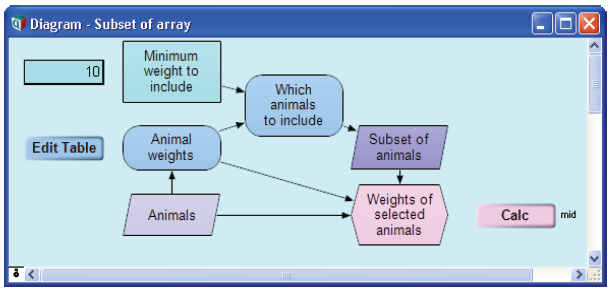Swapping y and x-index: This model swaps a computed or one-dimensional table value with its index, thereby making the computed value an index.

Use of MDTable: This model demonstrates the use of the MdTable function, which converts records in table form into multi-dimensional arrays. Multi-dimensional arrays are often useful for visualizing large sets of records. By allowing data to be viewed either as a graph or in a pivot table, the geometric relationship between records often becomes immediately evident.

## Optimizer Examples

This folder contains examples of how to use Analytica with Optimizer. These models are fully functional only if you have purchased the Optimizer license along with Analytica.

Airline NLP: This model gives examples of nonlinear programming optimization and Intelligent Arrays. The examples are:

• Simple airline decision problem to select the number of planes and fares to maximize profit; parametric analysis with respect to demand.
• The same problem with uncertainty, to maximize expected profit.
• The same problem with uncertainty, to maximize profit, given value of uncertain variables
• A dynamic model optimization over multiple years
• A dynamic analysis with optimization in each year

Asset allocation: Given many possible investments with varying risk-versus-return trade-offs, one can often reduce risk through diversification. In the best case, investing in two assets with identical expected appreciation, r, but which are perfectly anti-correlated in their co-variation yields the expected rate of return with no risk. The more general problem is to select a portfolio that both maximizes return and minimizes risk. There are several possible formulations for this, and this model explores three:

1. Minimize variance (risk) subject to a given expected return.
2. Maximize return subject to a given variance (risk)
3. Balance risk and return by maximizing expected utility, given a person’s level of risk aversion.

Automobile Production: This model is a linear programming example, taken from “Quick Review of Linear Programming,” Management Science Techniques for Consultants, by M.A. Trick (1996). Its objectives show all the varies results from a linear program:

• The optimal solution
• The value of the objective function at the optimum
• The solution status
• The reduced costs (dual values for the variables) at the optimal solution
• The slack or surplus values for the constraints at the optimal solution
• The shadow prices or dual values for the constraints at the optimal solution
• The range over which the objective function coefficient can vary in the linear program without changing the optimal solution
• The range over which a right-hand-side coefficient can vary without changing the dual value (shadow price) of the optimal solution

Beer Distribution LP: Three stages of the model that is used in the Optimizer user guide. Georgraphically distributed production plants (breweries) ship product (beer) to various market regions.

Big Mac Attack: This model addresses the issue of meeting one’s daily dietary requirements at McDonalds. The objective can either be to minimize cost, total caloric intake, or total carbohydrates. The model allows you to solve this problem to result in a Continuous, Integer, or Binary solution. Selecting Continuous results in a computationally easier problem, but less realistic answer, since you cannot order 4.35 Big Macs.

Capital Investment: This model is an example of capital budgeting for four possible projects, where the objective is to decide which projects to choose in order to maximize the total return.

Infeasible: Diagnosing an infeasible set of linear constraints.

Labor production allocation: A linear program to determine which product variants to produce and how labor should be allocated among the various production steps based on the workers’ skill sets.

Magic Square: Simple demonstration of a grouped-integer domain. Fill in a square with the digits 1 through n2 such that the rows and columns all sum to the same value.

NLP with Jacobian: This model demonstrates the use of the Jacobian and gradient in a nonlinear optimization with constraints. When a Jacobian is available analytically, it can accelerate the optimization convergence. The example is a simple geometric problem. Given a set of intersecting circles (where the inter- section of all the circles is not empty), which point of those contained in all the circles is closest to a target point?

Optimal can dimensions: This model is a simple example of using the NLP Optimizer in Analytica Optimizer. The model computes the optimal dimensions for a cylindrical can that must hold a given volume. The optimal can has the minimal surface area (and thus, uses the minimum material).

Optimal Production Allocation: This model is a simple example of linear programming in Analytica Optimizer. An integrated circuit manufacturer produces several different IC products (chips). Each chip is created by a sequence of processes, each carried out by a different machine. Every chip must pass through every process, but the time required for each process depends on the product. Some products require a lot of time at Process1, while other require very little time there but more time at other processes. The company’s objective is to determine how much of each product to produce to maximize profit without exceeding the capacity of each process.

Plane allocation LP: Allocate planes to origin/destination legs to maximize gross margin.

Polynomial NLP: A very simple 1-D example illustrating local the problem of local optima.

Problems with Local Optima: Nonlinear optimization problems often contain local optima as well as global optima. Ideally, we hope that an optimization algorithm would always find the global optima, but no algorithm can guarantee this in the general case. Local minima generally appear to have all the characteristics of a global optima, so that when the Optimizer has found a local minima, it usually terminates the search. The optional «guess» parameter to NlpDefine() provides a way to seed the search to the general area of where you think the global optima might be. When the Optimizer converges to a local optima, it is likely to be in the vicinity of the guess. So by trying a variety of guesses, you might be able to locate a set of local minima. Using the best of those might increase your chances of finding the true global optima. This model demonstrates this method.

Production Planning LP: This model is an example of production planning linear optimization. A company manufactures four versions of the same product and in the final part of the manufacturing process there are assembly, polishing, and packing operations. For each version, the time required for these operations is different, as is the profit per unit sold. How many of each variant should the company make per year and what is the associated profit?

Solve using NLP : This model demonstrates how an nonlinear programming formulation can be used to solve a non- linear equation. In this case, the equation is encoded as a constraint (this can be generalized to a system of nonlinear constraints), and the objective function is ignored (constant).

Sudoku with Optimizer: Find a solution to a Sudoku puzzle. Sudoku is a regular feature in many newspapers.

Traveling salesman: Find a minimal length tour through a set of cites. The problem is often cited as an example of an NP-complete problem, i.e., a problem solution can be verified in polynomial time, but cannot always be solved in reasonable (polynomial) time assuming, as is widely believed, that P ≠ NP .

Two Mines Model: This model is another production example. The Two Mines Company owns two different mines that produce an ore which, after being crushed, is graded into three classes: high, medium and low grade. The company has contracted to provide a smelting plant with 12 tons of high-grade, 8 tons of medium-grade, and 24 tons of low-grade ore per week. The two mines have different operating characteristics, in terms of cost to operate and production of each type of ore. How many days per week should each mine be operated to fulfill the smelting plant contract?

Vacation plan with PWL tax.ana: This example demonstrates the use of a piecewise-linear (PWL) relationship in a linear program. Although a piecewise-linear constraint is not linear, the problem can still be captured as an integer LP when you use the LinearInterp( ) function to encode the PWL,

## Risk Analysis

This folder contains applications relating to the field of risk analysis.

Earthquake expense risk: This model projects cost assessments of damage resulting from large earthquakes over time. It demonstrates risk analysis with time-dependence and costs of events shifted over time.

Seat belt safety: This model compares the value of various policies for restraints on occupants of automobiles.

Txc: This model demonstrates risk/benefit analysis, in this case regarding the benefits of reducing the emissions of fictitious air pollutant TXC.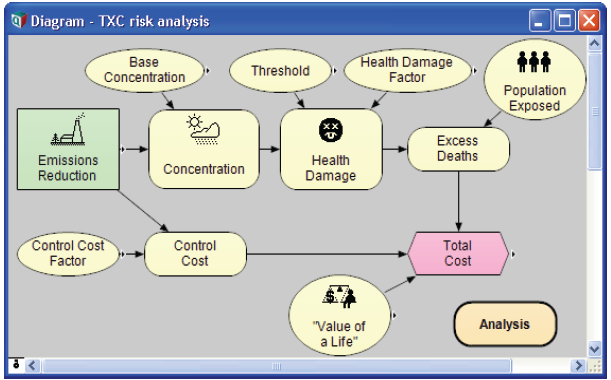## Tutorial Models

This folder contains stage-by-stage models from Analytica Tutorials.

Two models, Rent vs Buy Model and Rent vs Buy Analysis, are used in the Open a model to browse, Reviewing a model, and Analyzing a model tutorials. This model can be played in Analytica Cloud Player.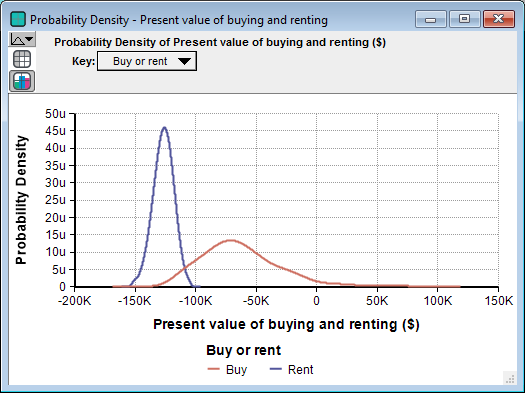Three models, Car cost, Car cost model ch 4 and Car cost model ch 5, walk through the Create a model and Arrays tutorials.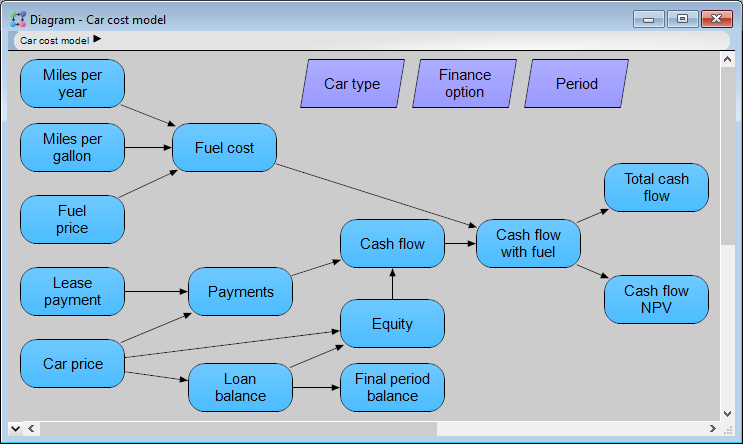Three models, Hares sub-module - act I, Foxes and hares sub-modules - act II and Foxes and hares act III, walk through the dynamic system model tutorial, which teaches the user about creating a Dynamic model. This set of models describes the dynamics of a predator/prey relationship. The completed model can be played in Analytica Cloud Player.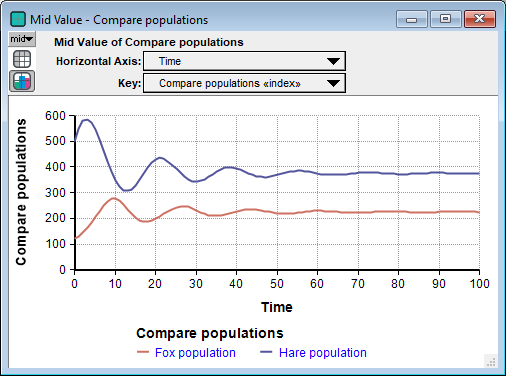## User Guide Examples

This folder contains the examples that are given in the Analytica User Guide.

### Basics

Expression Examples: The examples in this model demonstrate the building blocks for creating and editing variable definitions — expressions, standard operators, and mathematical functions. This model is used in the Analytica User Guide chapter Expressions.

Input and Output Nodes: This model is used in the Analytica User Guide chapter Creating Interfaces for End Users.

### Arrays and indexes

Array Examples: The examples in this model demonstrate the basics of working with multidimensional arrays. This model is used in the Analytica User Guide chapter Arrays and Indexes.

Array Function Examples: The examples in this model demonstrate many more of Analytica’s built-in array functions. This model is used in the Analytica User Guide chapter Array functions.

### Uncertainty and distributions

Analyzing Unc & Sens: The examples in this model demonstrate Analytica’s tools for analyzing the uncertainty of variables, relationships between uncertain variables, and sensitivity of outputs to changes in inputs. These include statistical functions and sensitivity analysis functions. This model is used in the Analytica User Guide chapter Statistics, Sensitivity, and Uncertainty Analysis.

Continuous Distributions: A continuous distribution is one that is defined for a continuous variable — that is, for a real-valued variable. The examples in this model demonstrate Analytica’s built-in functions that create or modify continuous distributions. This model is used in the Analytica User Guide chapter Probability Distributions.

Discrete Distributions: A discrete distribution is a probability distribution for a variable that can result only in certain, discrete outcomes. The examples in this model demonstrate Analytica’s built-in functions that create or evaluate discrete distributions. This model is used in the Analytica User Guide chapter Probability Distributions.

### Dynamic Models

A dynamic model is a model with one or more dynamic variables — that is, variables that can change over time. These models illustrate various uses of the Dynamic() function.

These models are used in the Analytica User Guide chapter Dynamic Simulation.

Dynamic & Dependencies: This model is a dynamic model that finds the downward velocity and position of a dropped object over a six second time period.

Dynamic & Uncertainty : This model shows three ways to use uncertainty with the Dynamic() function. In the first case, uncertainty samples are calculated once, at the initial time period. In the other two cases, new uncertainty samples are created for each time period (i.e., the values are re-sampled).

Dynamic Example 1: This model is the most simple dynamic model, with one variable that changes over time. This example finds the gasoline price for each of five years, assuming a 5% growth rate.

Dynamic Example 2 : This model is a slight increase in complexity over Dynamic Example 1. This model still uses one variable that changes over time. However, instead of assuming a fixed inflation rate, this example, looks at the price with three different inflation rates for comparison.

Dynamic on multiple indexes: Illustrates a dynamic loop involving simultaneous recurrences over two distinct indexes.

Dynamic on non-Time index: Use of the Dynamic() function to model a recurrence over an index other than Time.

## Libraries

The libraries in this folder contain functions that can be added to your model and used similarly to Analytica’s built-in functions. These libraries can be added to your model; Adding library to a model in the Analytica User Guide for information on how to add a library to a model.

Base conversion library: Functions that convert between binary, octal, decimal integer and hexadecimal values.

Bayes Function: This library contains Posterior(), a function for calculating posterior probabilities using Bayes’ Theorem.

Complex Library: This is a library of functions for working with complex numbers. It contains functions for basic arithmetic, polar representations of complex numbers, scalar functions for finding complex roots, logs, exponents, matrix functions, and trigonometric functions. Addition, subtraction, and scalar multiply are performed with the usual operators. Complex multiplication and complex division require the use of explicit functions. Complex numbers as seen by users of this library should always be in the Euclidean complex form, such as a + bi, where a is the real part and b is the imaginary part.

Concatenation: This library has been deprecated by extensions to the built-in Concat() and ConcatRows() functions, but is still included for backward compatibility for models that make use of its functions. This library contains functions to make concatenation more convenient. Functions Concat3() through Concat10() are generalizations of the built-in Concat() function which concatenate from 3 to 10 arrays in a single call (the built-in Concat() function concatenates two arrays). ConcatRows() concatenates all the rows of a single array.

Data Statistics Library:This library contains functions for calculating statistical quantities for a list of numbers over an explicit index other than Run, such as the index used for the statistics results in Analytica: the mean, variance, standard deviation, kurtosis, skewness, fractiles, covariance, correlation, frequency, etc.

Distribution Densities: The functions in this library return the probability densities (for continuous distributions) and prob- abilities (for discreet distributions) for the standard distribution functions that are built into Analytica. It also includes Cumulative distribution functions for continuous distributions and some discrete distributions, and inverse cumulative functions for most continuous distributions.

Distribution Variations:This library contains various functions for defining standard distributions using different sets of parameters.

Expand Index: This model contains the function Change Index. When this function is given an array indexed by one index, it returns an array indexed by another index. This model contains an example in which Change Index is used to combine cashflows over two different time periods into a single cashflow over a single time period.

Financial Library: This model contains a variety of corporate finance functions: Black-Scholes Option Values (Calloption, Putoption), Capital Asset Pricing Model (Capm), Miles/Ezzell Adjusted Cost of Capital (CostCapme), Modigliani/Miller Adjusted Cost of Capital (CostCapmm), Present Value of Perpetuity (Pvperp), Present Value of Growing Perpetuity (Pvgperp), and Weighted Average Cost of Capital (Wacc).

Flat File Library: This library provides functions for writing data to and from flat files, particularly between two- dimensional tables and comma-separated value (CSV) files.

Garbage Bin Library: This library provides a Recycle Bin for your model (including a recycle bin icon). To use it, simply drag your discarded objects into the recycle bin module. Unlike deletion, items in your recycle bin can be retrieved, and the Undo command (Control+Z) works for items dragged into the bin. If an item you put into the bin has dependents outside the bin, it shows arrows from the bin — a signal that you should either retrieve the item, since it is being used, or also drag its dependent(s) into the bin. To delete the items in the bin permanently, open the bin, select all its contents (with Control+A), and delete them (press Delete key).

Generalized Regression : Functions for Logistic, Probit and Poisson regression. While the Regression() function is used to obtain a function that predicts the expected value of an output given a new data point, generalized regression functions fit a function that predicts the probability or probability distribution of an out- put given a new data point.

Linked List Library: This library contains routines for manipulating linked lists. The simplest linked list is just Null — a linked list with zero elements. Any other linked list is a reference to a record indexed by Linked_List (an index defined in this library) with each cell containing a reference, to the element, and a pointer to the remainder of the list. Linked lists are created and manipulated using functions in this library:

• Use the function LL_Push to build the list.
• After a list is built, the easiest way to use and view it is to convert it back to an array using LL_To_RArray. This reverses the order of items in the linked list (which has the last item “pushed” into the linked list as the first item in the list) so that the array has the items ordered the same as when they were added to the list.
• The function LL_To_Array returns an array with the items ordered the same way as the linked list (the last entered item is first in the list/array).
• Other functions provide the first item in the list, the Nth item in the list, the list length, and allow you to remove (pop) the first item in the list.

Multivariate Distributions Library: This library contains functions for creating several multivariate distributions:

ODBC-Library: This library provides additional functionality (ValList, InsertRecSql, WriteTableSql) for using ODBC access to databases. Note that using ODBC requires Analytica Enterprise.

Optimization functions :Simple implementations of Newton-Raphson techniques for GoalSeek and Solve. These can be used to solve for a single value or for a vector of values in a non-linear model, and can be used from any edition of Analytica. The algorithms are not nearly as sophisticated and robust as those in the Analytica Optimizer edition.

Performance Profiler: Use this library to see which variables and functions are taking most of the computation time when running your model. Consult the model’s description for an explanation of how to use the library.

Structured Optimization Tools: Functions that are useful when creating structured optimization models (require Analytica Optimizer). These include functions to set the decisions variables’ definitions to the solution of an optimization, or to restore the definitions to their original values.

Tip

The ODBC and Profiling libraries require Analytica Enterprise, or ADE. They do not work with other versions of Analytica. Structured Optimization Tools require Analytica Optimizer.

## Summary

If you have created a model that other Analytica users would benefit from, please send it to us for possible inclusion in future versions of the Example Models folder. Send your well-constructed, thoroughly documented models to: support@lumina.com

You can also submit example models to the Analytica wiki, a web application that allows member of the Analytica community to collaborate and share information.

Here you can view example models submitted by others and to submit your own examples, and download many additional example models not bundled with the Analytica installer.

Go to the Analytica wiki home page to find all sorts of information on Analytica including additional information on Analytica functions, frequently asked questions (FAQs) and their answers, guides to modeling, what’s new in Analytica, and much more.